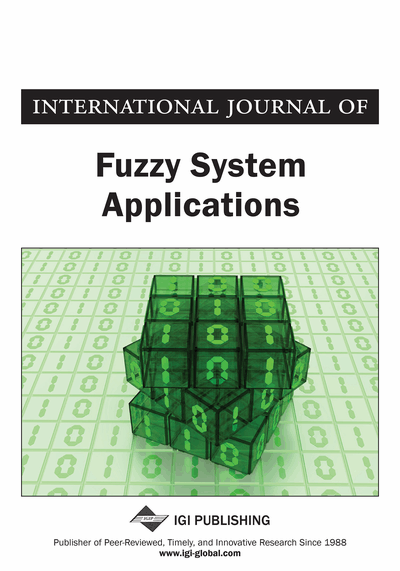# Approximate Fuzzy Continuity of Functions

Mark Burgin (University of California, USA) and Oktay Duman (TOBB Economics and Technology University, Turkey)
DOI: 10.4018/ijfsa.2011100103
Available
\$29.50
No Current Special Offers

## Abstract

The conventional continuity of a function was further advanced by the concept of approximate continuity introduced by Denjoy to solve some problems of differentiation and integration. According to this new type of continuity, the (classical) continuity conditions may be true not necessarily everywhere, but almost everywhere with respect to some measure, e.g., Borel measure or Lebesgue measure. However, functions that come from real life sources, such as measurement and computation, do not allow, in a general case, to test whether they are continuous or even approximately continuous in the strict mathematical sense. Hence, in this paper, the authors overcome these limitations by introducing and studying the more realistic concept of the approximate fuzzy continuity of functions.
Article Preview
Top

## Introduction

A natural definition of function continuity is based on the classical concept of sequence convergence. At the same time, there are more general concepts of sequence convergence. One of them is the concept of statistical convergence, introduced by Steinhaus (1951) and Fast (1951) and later reintroduced by Schoenberg (1959). Statistical convergence extends the concept of conventional convergence and is related to many useful constructions in series summability and probability theory.

It seems natural to try to extend the concept of continuity using statistical convergence instead of conventional convergence. However, this brings us back to the same concept of function continuity as the following explanation demonstrates.

Assume that a function f: RR is continuous at a point aR in the conventional sense. Then, it is well-known that f(x) is defined at a and for any sequence l = { aiR; i = 1, 2, 3, …} that converges to a, the point f(a) is the limit of the sequence{ f(ai) ∈ R; i = 1, 2, 3, …} or formally, for any sequence l = { aiR; i = 1, 2, 3, …}, the condition limi→∞ai = a implies limi→∞f(ai) = f(a). Using the statistical continuity instead of conventional continuity, we come to the following definition of statistical continuity. A function f: RR is statistically continuous at a point aR if f(x) is defined at a and for any sequence l = { aiR; i = 1, 2, 3, …} that converges to a, the point f(a) is the statistical limit of the sequence{ f(ai) ∈ R; i = 1, 2, 3, …}. Recall that a sequence l = {ai ; i = 1, 2, 3,…} is statistically convergent to a or a = stat-lim l if d({iN; |ai - a| ≥ ε }) = 0 for every ε > 0 where the asymptotic density d(K) of a set KN is equal to limn→∞ (1/n) |Kn| and Kn = {kK; kn} (Steinhaus, 1951; Fast, 1951; Schoenberg, 1959; Fridy, 1985). Thus, for a statistically continuous at a point a function f and any sequence l = { aiR; i = 1, 2, 3, …} that converges to a, we have that either the sequence{ f(ai) ∈ R; i = 1, 2, 3, …} converges to f(a) or there are infinitely many elements ai in l such that | f(ai) - f(a) | ≥ ε for some ε. If the function f is statistically continuous at the point a but not continuous at this point, then there is a sequence l such that there are infinitely many elements aij in l such that | f(aij) - f(a) | ≥ ε. Let us take all these elements aij. They form a sequence h = { aijR; j = 1, 2, 3, …} and | f(aij) - f(a) | ≥ ε for all aij, j = 1, 2, 3, … . By the definition of the asymptotic density d(K), we have that d({iN; |aij - a| ≥ ε }) = 1. However, as lim l = a, it has to be d({iN; |aij - a| ≥ ε }) = 0. This contradiction shows that any sequence{ f(ai) ∈ R; i = 1, 2, 3, …} converges to f(a) when the sequence l = { aiR; i = 1, 2, 3, …} that converges to a. Consequently, f is a continuous function in the conventional sense, i.e., the new approach does extend the conventional definition of function continuity.

## Complete Article List

Search this Journal:
Reset
Volume 12: 1 Issue (2023)
Volume 11: 4 Issues (2022)
Volume 10: 4 Issues (2021)
Volume 9: 4 Issues (2020)
Volume 8: 4 Issues (2019)
Volume 7: 4 Issues (2018)
Volume 6: 4 Issues (2017)
Volume 5: 4 Issues (2016)
Volume 4: 4 Issues (2015)
Volume 3: 4 Issues (2013)
Volume 2: 4 Issues (2012)
Volume 1: 4 Issues (2011)
View Complete Journal Contents Listing